Test: Engineering Mathematics- 3

# Test: Engineering Mathematics- 3

Test Description

## 10 Questions MCQ Test GATE Computer Science Engineering(CSE) 2023 Mock Test Series | Test: Engineering Mathematics- 3

Test: Engineering Mathematics- 3 for Computer Science Engineering (CSE) 2023 is part of GATE Computer Science Engineering(CSE) 2023 Mock Test Series preparation. The Test: Engineering Mathematics- 3 questions and answers have been prepared according to the Computer Science Engineering (CSE) exam syllabus.The Test: Engineering Mathematics- 3 MCQs are made for Computer Science Engineering (CSE) 2023 Exam. Find important definitions, questions, notes, meanings, examples, exercises, MCQs and online tests for Test: Engineering Mathematics- 3 below.
Solutions of Test: Engineering Mathematics- 3 questions in English are available as part of our GATE Computer Science Engineering(CSE) 2023 Mock Test Series for Computer Science Engineering (CSE) & Test: Engineering Mathematics- 3 solutions in Hindi for GATE Computer Science Engineering(CSE) 2023 Mock Test Series course. Download more important topics, notes, lectures and mock test series for Computer Science Engineering (CSE) Exam by signing up for free. Attempt Test: Engineering Mathematics- 3 | 10 questions in 30 minutes | Mock test for Computer Science Engineering (CSE) preparation | Free important questions MCQ to study GATE Computer Science Engineering(CSE) 2023 Mock Test Series for Computer Science Engineering (CSE) Exam | Download free PDF with solutions
 1 Crore+ students have signed up on EduRev. Have you?
Test: Engineering Mathematics- 3 - Question 1

### The matrix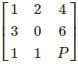has one eigenvalue equal to 3. The sum of the other two eigenvalues is

Detailed Solution for Test: Engineering Mathematics- 3 - Question 1

Sum of the eigen values of matrix is = Sum of diagonal values present in the matrix

∴ 1 + 0 + P = 3 + λ+ λ3

⇒ P + 1 = 3 + λ+ λ3

⇒ λ+ λ= P + 1 – 3 = P – 2

Test: Engineering Mathematics- 3 - Question 2

### What is the determinant of matrix X if 4 and (2 + 7i) are the eigenvalues of X where i = √−1?

Detailed Solution for Test: Engineering Mathematics- 3 - Question 2

Two eigen value of X is 4 and (2 + 7i)

∴ (2 - 7i) (conjugate roots) must be the third root

Determinant of P = product of eigenvalues

Δ = 4 × (2 + 7i) × (2 - 7i)

Δ = 212

Test: Engineering Mathematics- 3 - Question 3

### In the given matrix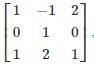one of the eigenvalues is 1. The eigenvectors corresponding to the eigenvalue 1 are

Detailed Solution for Test: Engineering Mathematics- 3 - Question 3

For a given matrix A if V is the eigen vector corresponding to the eigen value λ, then:

AV = λV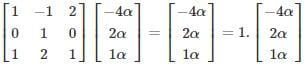∴ {α (−4, 2, 1) |α ≠ 0, αϵR} are the corresponding eigenvectors.

Test: Engineering Mathematics- 3 - Question 4

Which of the below-given statements is/are true?

I. The eigenvalue of the lower triangular matrix is just the diagonal elements of the matrix.

II. The product of the eigenvalue of a matrix is equal to its trace.

III. If 1/λ is an eigenvalue of A’(inverse of A) then orthogonal of A also have 1/λ as its eigenvalue.

Detailed Solution for Test: Engineering Mathematics- 3 - Question 4
• The eigenvalue of the triangular (lower or upper) matrix are just the diagonal elements of matrix.
• The product of the eigenvalue of a matrix is equal to its determinants.
• If λ is the eigenvalue of matrix, then 1/λ is the eigenvalue of its inverse since orthogonal is equal to inverse matrix then it has 1/λ as its eigenvalue

​Example: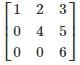Eigenvalues are 1, 4 and 6 (diagonal elements)

Product of eigen value = determinants = 1 × 4 × 6 = 24

Orthogonal matrix and Inverse of given matrix have eigenvalues: 1,1/4 and 1/6

Test: Engineering Mathematics- 3 - Question 5

Consider the following 2 × 2 matrix A where two elements are unknown and are marked by a and b. The eigenvalues of this matrix are - 1 and 7. What are the values of a and b?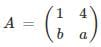Detailed Solution for Test: Engineering Mathematics- 3 - Question 5

W.K.T.∑λ= ∑aii
∴ - 1 + 7 = 1 + a ⇒ a = 5

Also π λi = |A|

∴ - 1 × 7 = a – 4b

- 7 = 5 – 4b ⇒ b = 3

Test: Engineering Mathematics- 3 - Question 6

Let A be the 2 X 2 matrix with elements a11 = 2, a12 = 3, a21 = 1 and a22 = 4 then the eigenvalues of the Matrix are A5?

Detailed Solution for Test: Engineering Mathematics- 3 - Question 6

Given Matrix: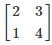Characteristic Equation: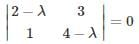(2−λ) (4−λ) −3 = 0

λ2 − 6λ + 5 = 0

λ = 5 or λ = 1

*Answer can only contain numeric values
Test: Engineering Mathematics- 3 - Question 7

The latent values of the matrix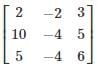are 1, 1, 2 and the number of linearly independent latent vectors for the repeated root 1 is –

Detailed Solution for Test: Engineering Mathematics- 3 - Question 7

Eigen vectors are also called invariant vectors, characteristic vectors, or latent vectors. Eigenvalues are also called characteristic roots or latent roots.

(A - λI)X = 0

Given λ = 1

⇒ (A - I) X = 0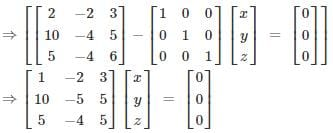⇒ x = 2y + 3x = 0, 10x – 5y + 5z = 0, 5x – 4y + 5z = 0

By solving above equations, we get x =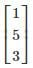i.e. one linearly independent latent vector.

*Answer can only contain numeric values
Test: Engineering Mathematics- 3 - Question 8

Consider a Matrix M = uTvT where u =  (112) and v =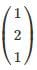also uT denotes the transpose of matrix u. Find the largest eigenvalue of M?

Detailed Solution for Test: Engineering Mathematics- 3 - Question 8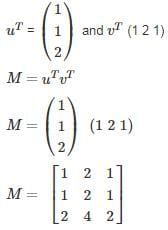Characteristic equation is given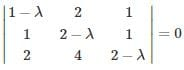λ3 − 5λ2 = 0

λ = 0 or λ = 5

Therefore largest value is 5.

Test: Engineering Mathematics- 3 - Question 9

What is the absolute difference of the eigenvalues for the matrix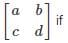ad – bc = 6 and a + d = 7?

Detailed Solution for Test: Engineering Mathematics- 3 - Question 9

Let λ1 and λ2 be the two eigen values

Product of eigen value is equal to determinant of matrix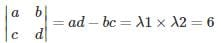Sum of eigen value is equal to its trace

a + d = λ1 + λ2 = 7

λ1 × (7 − λ1) = 6

λ12 − 7λ + 6 = 0

λ1 = 6 or λ2 = 1

|λ1 − λ2| = 5

Test: Engineering Mathematics- 3 - Question 10

Consider the matrix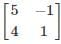which one of the following statements is TRUE for the eigenvalues and eigenvectors of the matrix?

Detailed Solution for Test: Engineering Mathematics- 3 - Question 10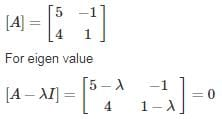(5 - λ) (1 - λ) + 4 = 0

5 – 5λ - λ + λ2 + 4 = 0

λ2 - 6λ + 9 = 0

(λ - 3)2 = 0

λ = 3

for eigen vector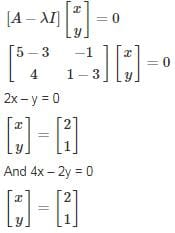So, only one independent eigen vector.

## GATE Computer Science Engineering(CSE) 2023 Mock Test Series

150 docs|215 tests
 Use Code STAYHOME200 and get INR 200 additional OFF Use Coupon Code
Information about Test: Engineering Mathematics- 3 Page
In this test you can find the Exam questions for Test: Engineering Mathematics- 3 solved & explained in the simplest way possible. Besides giving Questions and answers for Test: Engineering Mathematics- 3, EduRev gives you an ample number of Online tests for practice

## GATE Computer Science Engineering(CSE) 2023 Mock Test Series

150 docs|215 tests